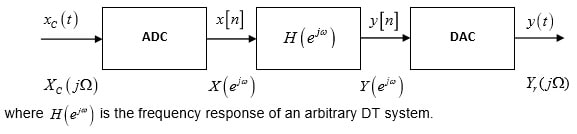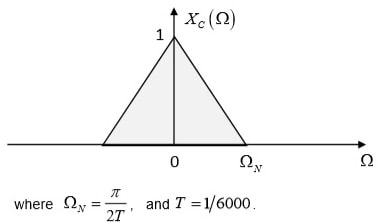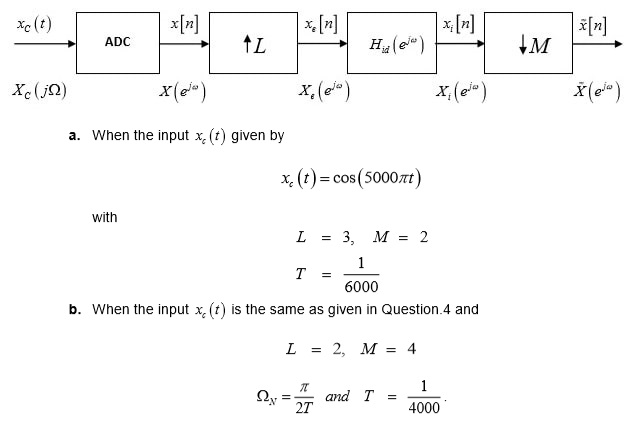# Discrete Signal processing assignment 1

1. Describe the following block diagram without any knowledge about the input. i.e. state what is going to happen at each of the stages. Also list the key block of the ADC and DAC.2. In the figure shown above, describe the first analog-to-digital block, express the output of the first block in terms of the input mathematically (in time as well as frequency domain).

3. For the figure given above express the output y(t) in terms of the input xCt (mathematically) with, H(e) = 2 ∀ ω .

4. Graphically illustrate the effect of ADC and DAC conversion when H(e) = 1 with the input given below.5. Graphically illustrate the effects of the processes shown in the figure below